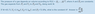# Finding area from work, pressure and volume

JoeyBob
Homework Statement:
see attached
Relevant Equations:
W=integral(PdV)
So I basically took the integral and ended up with W=PVf-A(Vf^3)/3-PVi+A(Vf^3)/3

so 65.7=72*5.3-A(5.3)^3/3-72(2.4)+A(2.4)^3/3

But when I solve for A I get the wrong answer of 3.179 when the answer is suppose to be 5.05. I've checked my calculation with an algebra calculator too...

#### Attachments

•question.PNG
5.4 KB · Views: 57

Homework Helper
Gold Member
2022 Award
Homework Statement:: see attached
Relevant Equations:: W=integral(PdV)

So I basically took the integral and ended up with W=PVf-A(Vf^3)/3-PVi+A(Vf^3)/3

so 65.7=72*5.3-A(5.3)^3/3-72(2.4)+A(2.4)^3/3

But when I solve for A I get the wrong answer of 3.179 when the answer is suppose to be 5.05. I've checked my calculation with an algebra calculator too...
You seem to have mixed up P0 and P1.

JoeyBob
You seem to have mixed up P0 and P1.
Wait wouldn't tha mean there's two unknowns then? How would I find P0?

Homework Helper
Gold Member
2022 Award
Wait wouldn't tha mean there's two unknowns then? How would I find P0?
You have two equations relating P0, P1, P2, V1, V2 and A.

JoeyBob
You have two equations relating P0, P1, P2, V1, V2 and A.

Wait so is it P1=P0+AV and then the integral eqn?

Homework Helper
Gold Member
2022 Award
Wait so is it P1=P0+AV and then the integral eqn?
No.
You are given P=P0-AV2 as a general fact. In particular this will be true at the initial and final states. Plug in the values/variables for those states to get two equations.

•Delta2
Mentor
You have $$P_1=P_0-AV_1^2$$ so $$P_0=P_1+AV_1^2$$So you can eliminate ##P_0##

Homework Helper
Gold Member
2022 Award
Well the problem statement says: $$P=P_0-AV^2$$so at the initial condition, $$P_1=P_0-AV_1^2$$So my algebra tells me that $$P_0=P_1+AV_1^2$$
What am I missing?
Sorry, I read what I was expecting, not what you wrote.
I read your second line as $$P_2=P_0+AV_2^2$$, reinforced by your comment about eliminating P0. So what I should have written is $$P_2=P_0-AV_2^2$$.

Mentor
Sorry, I read what I was expecting, not what you wrote.
I read your second line as $$P_2=P_0+AV_2^2$$, reinforced by your comment about eliminating P0. So what I should have written is $$P_2=P_0-AV_2^2$$.
What do you need to know P2 for?